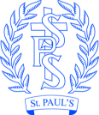# Monday 29th June

 Maths LO: I can find a unit fraction of a shape Activity This week we are going to revisit fractions. Today we will be learning about unit fractions. The number of equal parts is the denominator of the fraction. This number goes below the line. The numerator is the part of the fraction that we are looking at / colouring. This goes above the line. A fraction where the numerator is 1 is called a unit fraction. For example;Find a picture of these countries flags. What fraction is each stripe? Poland Italy Romania Ukraine Bulgaria Using your paper shapes can you fold, draw the lines on to show the fractions and shade the following: ½ of a square 1/3 of a rectangle ¼ of a circle 1/3 of a square ¼ of a rectangle ½ of a circle Remember each fraction needs to be equal. Looking for more: Can any of these shapes be folded in a different way to show any of the fractions? Resources: Pictures of flags – you could search for them on swiggle.org.uk with grown up supervision. Paper cut into shapes, 2 square, 2 rectangles, 2 circles.